SVG蒙版功能可将蒙版应用于SVG形状。蒙版可确定SVG形状的哪些部分可见，以及具有什么透明度。运行效果可以将SVG蒙版视为剪切路径的更高级版本。

# 一、简单的蒙版

``````<svg width="500" height="120">
<defs>
<rect x="0" y="0" width="100" height="50" style="stroke:none; fill: #ffffff" />
</defs>
<rect x="1" y="1" width="100" height="100" style="stroke: none; fill: #0000ff; mask: url(#mask1)" />
</svg>
``````

# 二、其他形状的蒙版

``````<svg>
<defs>
<circle cx="25" cy="25" r="25" style="stroke:none; fill: #ffffff"/>
</defs>

<rect x="1" y="1" width="100" height="100"

</svg>
``````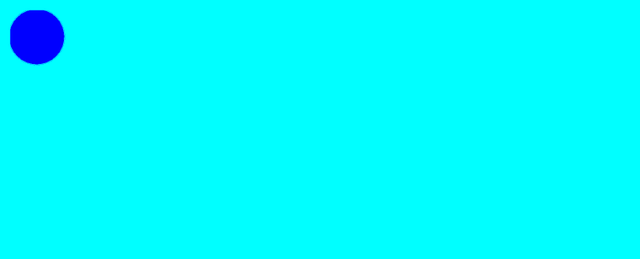# 2. 案例

``````<svg width="500" height="120">
<defs>

<rect x="0" y="0"  width="100" height="50"
style="stroke:none; fill: #ffffff"/>

<rect x="0" y="50" width="100" height="50"
style="stroke:none; fill: #666666"/>
</defs>

<text x="10" y="55" style="stroke: none; fill: #000000;">
此文本在矩形下方
</text>

<rect x="1" y="1" width="100" height="100"
</svg>
``````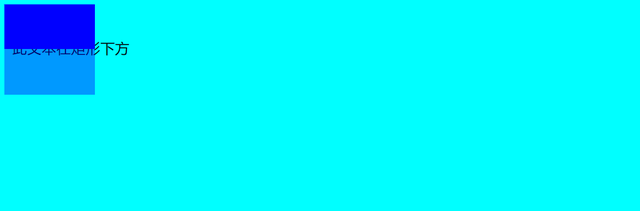# 四、在蒙版中使用渐变

``````<svg width="500" height="120">
<defs>
<stop offset="0%" stop-color="#ffffff" stop-opacity="1" />
<stop offset="100%" stop-color="#000000" stop-opacity="1" />

<rect x="0" y="0" width="200" height="100" style="stroke:none; fill: url(#gradient1)" />
</defs>

<text x="10" y="55" style="stroke: none; fill: #000000;">

</text>
<rect x="1" y="1" width="200" height="100" style="stroke: none; fill: #FF0000; mask: url(#mask4)" />
</svg>
``````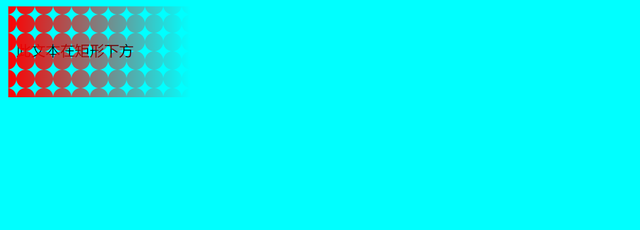SVG代码：

``````<svg width="500" height="120">
<defs>

x1="0%"   y1="0%"
x2="100%" y2="0%"
<stop offset="0%"   stop-color="#ffffff" stop-opacity="1"/>
<stop offset="100%" stop-color="#000000" stop-opacity="1"/>

<pattern id="pattern2"
x="10" y="10" width="20" height="20"
patternUnits="userSpaceOnUse" >

<circle cx="10" cy="10" r="10" style="stroke: none; fill: #FF0000; " />

</pattern>

<rect x="0" y="0"  width="200" height="100"
</defs>

<text x="10" y="55" style="stroke: none; fill: #000000;">
此文本在矩形下方
</text>
<rect x="1" y="1" width="200" height="100"
</svg>
``````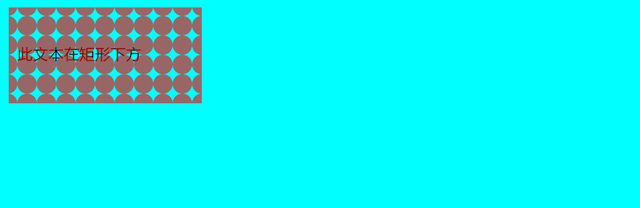# 五、在蒙版中使用填充图案

``````<svg width="500" height="120">
<defs>
<pattern id="pattern1"
x="10" y="10" width="20" height="20"
patternUnits="userSpaceOnUse" >

<circle cx="10" cy="10" r="10" style="stroke: none; fill: #999999" />
</pattern>

<rect x="0" y="0"  width="200" height="100"
style="stroke:none; fill: url(#pattern1)"/>
</defs>

<text x="10" y="55" style="stroke: none; fill: #000000;">
此文本在矩形下方
</text>
<rect x="1" y="1" width="200" height="100"
</svg>
``````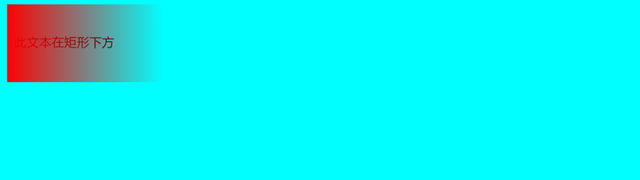# 六、总结